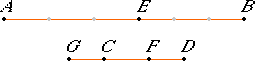# Proposition 5

If a magnitude is the same multiple of a magnitude that a subtracted part is of a subtracted part, then the remainder also is the same multiple of the remainder that the whole is of the whole.

Let the magnitude AB be the same multiple of the magnitude CD that the subtracted part AE is of the subtracted part CF.

I say that the remainder EB is also the same multiple of the remainder FD that the whole AB is of the whole CD.

Make CG so that EB is the same multiple of CG that AE is of CF.V.1

Then, since AE is the same multiple of CF that EB is of GC, therefore AE is the same multiple of CF that AB is of GF.

But, by the assumption, AE is the same multiple of CF that AB is of CD.

Therefore AB is the same multiple of each of the magnitudes GF and CD. Therefore GF equals CD.

Subtract CF from each. Then the remainder GC equals the remainder FD.

And, since AE is the same multiple of CF that EB is of GC, and GC equals DF, therefore AE is the same multiple of CF that EB is of FD.

But, by hypothesis, AE is the same multiple of CF that AB is of CD, therefore EB is the same multiple of FD that AB is of CD.

That is, the remainder EB is the same multiple of the remainder FD that the whole AB is of the whole CD.

Therefore, If a magnitude is the same multiple of a magnitude that a subtracted part is of a subtracted part, then the remainder also is the same multiple of the remainder that the whole is of the whole.

Q.E.D.

## Guide

This proposition is analogous to V.1 but involves differences rather than sums. It states that multiplication by numbers distributes over subtraction of magnitudes: m (x – y) = mx – my.

Note that all the magnitudes in this proposition are of the same kind.

#### The problem of parts

There’s a construction at the beginning of the proof to make a part of a magnitude:
Make CG so that EB is the same multiple of CG that AE is of CF.
so that, if for example, AE is a third of CF, then CG is to be made a third of EB. Such constructions cannot be made for all kinds of magnitudes, in particular, angles and arcs.

Alternative proofs that don’t require constructions of parts are relatively easy to find. A more interesting problem of general constructions for magnitudes is discussed in the Guide for proposition V.18.

This proposition is not used in the rest of the Elements.